# Mathematics Grade 5 by Siyavula Uploader - HTML preview

PLEASE NOTE: This is an HTML preview only and some elements such as links or page numbers may be incorrect.
Download the book in PDF, ePub, Kindle for a complete version.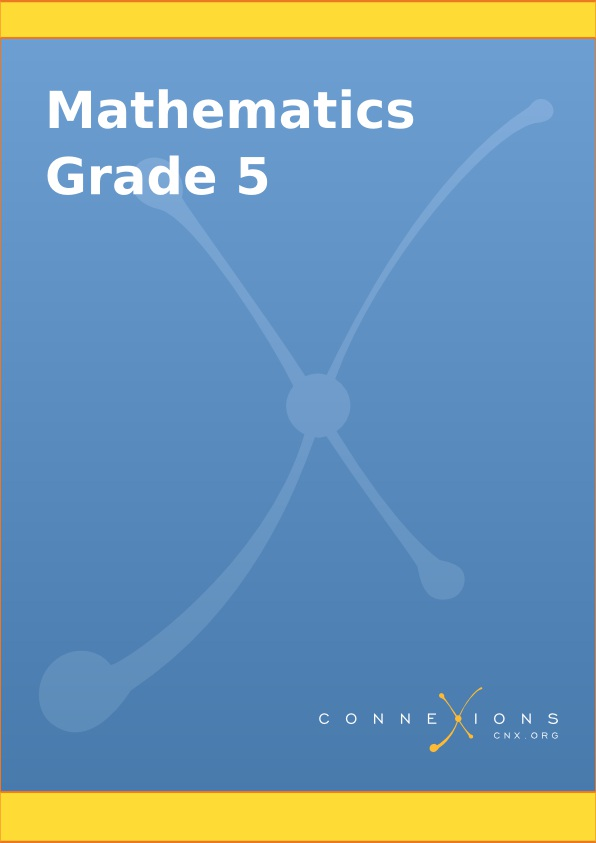Mathematics Grade 5

Collection edited by: Siyavula Uploader

Content authors: Siyavula Uploader and Siyavula Uploader

Online: < http://cnx.org/content/col10994/1.3> This selection and arrangement of content as a collection is copyrighted by Siyavula Uploader.

It is licensed under the Creative Commons Attribution License: http://creativecommons.org/licenses/by/3.0/

Collection structure revised: 2009/09/23

For copyright and attribution information for the modules contained in this collection, see the " Attributions" section at the end of the collection.

Mathematics Grade 5

Table of Contents

Chapter 1. Term 1

1.1. To be able to do mental arithmetic

MATHEMATICS

Number Concept, Addition and Subtraction

Subtraction

EDUCATOR SECTION

Memorandum

Leaner Section

Content

Activity: To be able to do mental arithmetic [LO 1.9]

Assessment

1.2. To be able to solve problems in context

MATHEMATICS

Number Concept, Addition and Subtraction

Subtraction

EDUCATOR SECTION

Memorandum

Leaner Section

Content

Activity: To be able to solve problems in context [LO 1.6]

To use a series of techniques to do calculations [LO 1.10]

Assessment

1.3. To determine the equivalence and validity of different representations

MATHEMATICS

Number Concept, Addition and Subtraction

Subtraction

EDUCATOR SECTION

Memorandum

Content

Activity: To determine the equivalence and validity of different

representations [LO 2.6]

To use strategies to check solutions [LO 1.11]

Assessment

1.4. To use a series of techniques to do calculations

MATHEMATICS

Number Concept, Addition and Subtraction

Subtraction

EDUCATOR SECTION

Memorandum

PUZZLE

Content

Activity: To be able to solve problems in context [LO 1.10]

To use strategies to check solutions [LO 1.11]

Assessment

1.5. To solve problems in context

MATHEMATICS

Number Concept, Addition and Subtraction

Subtraction

EDUCATOR SECTION

Memorandum

Leaner Section

Content

Activity: To solve problems in context [LO 1.6]

TEST

Assessment

1.6. To be able to do mental arithmetic

MATHEMATICS

Number Concept, Addition and Subtraction

EDUCATOR SECTION

Memorandum

Leaner Section

Content

ACTIVITY: To be able to do mental arithmetic [LO 1.9]

Assessment

1.7. To count and calculate correctly without using a pencil and paper

MATHEMATICS

Number Concept, Addition and Subtraction

EDUCATOR SECTION

Memorandum

Leaner Section

Content

ACTIVITY: To count and calculate correctly without using a pencil and paper

[LO 1.1, LO 1.8]

Assessment

1.8. To recognise numbers and represent them correctly

MATHEMATICS

Number Concept, Addition and Subtraction

EDUCATOR SECTION

Memorandum

Leaner Section

Content

Activity: To recognise numbers and represent them correctly [LO 1.3]

To recognise the place value of digits [LO 1.4]

Assessment

1.9. To improve your mental arithmetic skills

MATHEMATICS

Number Concept, Addition and Subtraction

EDUCATOR SECTION

Memorandum

Content

ACTIVITY: To improve your mental arithmetic skills [LO 1.9]

To examine and extend numeric patterns [LO 2.1]

Assessment

1.10. To recognise numbers and compare them to one another

MATHEMATICS

Number Concept, Addition and Subtraction

EDUCATOR SECTION

Memorandum

Answer on p. 11-12

Leaner Section

Content

Activity: To recognise numbers and compare them to one another [LO 1.3]

To be able to calculate correctly [LO 1.8]

DO YOU REMEMBER THIS?

Assessment

1.11. To represent, recognise and compare numbers

MATHEMATICS

Number Concept, Addition and Subtraction

EDUCATOR SECTION

Memorandum

Leaner Section

Content

Activity: To represent, recognise and compare numbers [LO 1.3]

To be able to calculate correctly [LO 1.8]

To improve mental arithmetic skills [LO 1.9]

CHALLENGE

PLAY THIS GAME

TIME FOR SELF-ASSESSMENT ONCE MORE

Assessment

1.12. To count forward in intervals

MATHEMATICS

Number Concept, Addition and Subtraction

EDUCATOR SECTION

Memorandum

Leaner Section

Content

Activity: To count forward in intervals [LO 1.1]

To recognise, represent, describe and compare numbers, counting down and up

in fixed intervals [LO 1.3]

TEST

Assessment

1.13. To be able to count correctly

MATHEMATICS

Number Concept, Addition and Subtraction

Addition

EDUCATOR SECTION

Memorandum

Content

Activity: To be able to count correctly [LO 1.1]

To be able to calculate correctly [LO 1.8]

To be able to do mental arithmetic [LO 1.9]

Assessment

1.14. To use a series of techniques to do calculations

MATHEMATICS

Number Concept, Addition and Subtraction

Addition

EDUCATOR SECTION

Memorandum

Content

Activity: To use a series of techniques to do calculations [LO 1.10]

Assessment

1.15. To describe and illustrate different cultures' ways of writing

MATHEMATICS

Number Concept, Addition and Subtraction

Addition

EDUCATOR SECTION

Memorandum

Brain Teaser

Content

Activity: To describe and illustrate different cultures' ways of writing [LO 1.2]

Assessment

1.16. To recognise, describe and use laws

MATHEMATICS

Number Concept, Addition and Subtraction

Addition

EDUCATOR SECTION

Memorandum

Content

Activity: To recognise, describe and use laws [LO 1.12]

DID YOU KNOW

Assessment

1.17. To estimate and calculate by selecting and using suitable operations

MATHEMATICS

Number Concept, Addition and Subtraction

Addition

EDUCATOR SECTION

Memorandum

Leaner Section

Content

Activity: To estimate and calculate by selecting and using suitable operations

[LO 1.8]

Assessment

1.18. To solve problems in context

MATHEMATICS

Number Concept, Addition and Subtraction

Addition

EDUCATOR SECTION

Memorandum

Leaner Section

Content

Activity: To solve problems in context [LO 1.6]

To use a series of techniques to do calculations [LO 1.10]

To use strategies to check solutions [LO 1.11]

Assessment

1.19. To determine the equivalence and validity of different representations

MATHEMATICS

Number Concept, Addition and Subtraction

Addition

EDUCATOR SECTION

Memorandum

Content

Activity: To determine the equivalence and validity of different representations

[LO 2.6]

To use strategies to check solutions [LO 1.11]

SELF-ASSESSMENT

BRAIN TEASER

Assessment

1.20. To use a series of techniques to do calculations

MATHEMATICS

Number Concept, Addition and Subtraction

Addition

EDUCATOR SECTION

Memorandum

Content

Activity: To use a series of techniques to do calculations [LO 1.10]

Assessment

1.21. To be able to do mental arithmetic

MATHEMATICS

Number Concept, Addition and Subtraction

Addition

EDUCATOR SECTION

Memorandum

Leaner Section

Content

Activity: To be able to do mental arithmetic [LO 1.9]

Assessment

1.22. To use techniques to do calculations

MATHEMATICS

Number Concept, Addition and Subtraction

Addition

EDUCATOR SECTION

Memorandum

Leaner Section

Content

Activity: To use techniques to do calculations [LO 1.10]

Assessment

1.23. To solve in context problems that concern economic issues

MATHEMATICS

Number Concept, Addition and Subtraction

Addition

EDUCATOR SECTION

Memorandum

Total: 20

Leaner Section

Content

Activity: To solve in context problems that concern economic issues [LO 1.6]

TEST

Assessment

1.24. To be able to do mental arithmetic

MATHEMATICS

Number Concept, Addition and Subtraction

Subtraction

EDUCATOR SECTION

Memorandum

Content

Activity: To be able to do mental arithmetic [LO 1.9]

Assessment

1.25. To describe and illustrate different cultures' ways of writing

MATHEMATICS

Number Concept, Addition and Subtraction

Subtraction

EDUCATOR SECTION

Memorandum

Content

Activity: To describe and illustrate different cultures' ways of writing [LO 1.2]

Assessment

1.26. To use a series of techniques to be able to do calculations

MATHEMATICS

Number Concept, Addition and Subtraction

Subtraction

EDUCATOR SECTION

Memorandum

Leaner Section

Content

Activity: To use a series of techniques to be able to do calculations [LO 1.10]

Assessment

1.27. To examine and expand numeric patterns

MATHEMATICS

Number Concept, Addition and Subtraction

Subtraction

EDUCATOR SECTION

Memorandum

Content

Activity: To examine and expand numeric patterns [LO 2.1]

Assessment

1.28. To determine the output values for given input values

MATHEMATICS

Number Concept, Addition and Subtraction

Subtraction

EDUCATOR SECTION

Memorandum

PUZZLE

Leaner Section

Content

Activity: To determine the output values for given input values [LO 2.3]

Assessment

1.29. To calculate by using selected computations

MATHEMATICS

Number Concept, Addition and Subtraction

Subtraction

EDUCATOR SECTION

Memorandum

Leaner Section

Content

Activity: To calculate by using selected computations [LO 1.8]

Assessment

Chapter 2. Term 2

2.1. Multiplication

MATHEMATICS

Grade 5

MULTIPLICATION AND DIVISION

Module 32

MULTIPLICATION

Activity 1:

To recognise and represent the multiples of single-digit numbers [LO 1.3.6]

Activity 2:

To recognise and represent the factors of at least any two-digit whole number

[LO 1.3.7]

Activity 3:

To be able to do mental arithmetic [LO 1.9.2]

Activity 4:

To describe and illustrate different ways of writing in different cultures [LO

1.2]

Activity 5:

To use a series of techniques to do computations with whole numbers [LO

1.10.4]

Activity 6:

To calculate by using selected computations [LO 1.9.2]

Activity 7:

To recognise and use the characteristics of multiplication with whole numbers

[LO 1.12.3]

Activity 8:

To estimate and calculate by means of rounding off [LO 1.8.1]

Activity 9 :

Activity 10:

Activity 11:

To use a series of techniques to do calculations [LO 1.10.5]

Activity 12:

To be able to do mental arithmetic [LO 1.9.2]

Activity 13:

To be able to solve problems in context [LO 1.6.1]

To be able to use a series of techniques to do calculations [LO 1.10.5]

Activity 14:

To determine the equivalence and the validity of different representations [LO

2.6.1]

To use strategies to check solutions [LO 1.11]

Activity 15:

To solve problems in context [LO 1.6.1]

Assessment

Memorandum

ANOTHER BRAIN-TEASER

2.2. Division

MATHEMATICS

Grade 5

MULTIPLICATION AND DIVISION

Module 33

DIVISION

Activity 1:

To be able to do mental arithmetic [LO 1.10]

Activity 2:

To recognise, describe and use the reciprocal relation between multiplication

and division

[LO 1.12.1]

Activity 3:

To recognise and represent numbers and to describe and compare them [LO

1.3]

Activity 4:

To describe and illustrate other cultures' ways of writing [LO 1.2]

Activity 5:

To describe observed patterns and rules in own terms [LO 2.2]

Activity 6:

To calculate by using selected computations [LO 1.8.4]

Activity 7:

To be able to do mental arithmetic [LO 1.9.2]

Activity 8:

To estimate and calculate by using selected computations [LO 1.8.5]

Activity 9:

To be able to solve problems in context [LO 1.6.1]

Activity 10:

To solve problems in context [LO 1.6]

Activity 11:

To determine the equivalence and validity of different representations of the

same problem by means of comparison and discussion [LO 2.6]

Activity 12:

To calculate by using selected computations [LO 1.8.5]

Assessment

Memorandum

Chapter 3. Term 3

3.1. To recognise, classify and represent fractions (positive numbers)

MATHEMATICS

Grade 5

ORDINARY AND DECIMAL FRACTIONS

Module 45

TO RECOGNISE, CLASSIFY AND REPRESENT FRACTIONS (POSITIVE

NUMBERS)

Activity 1:

To recognise, classify and represent fractions (positive numbers) in order to

describe and compare them [LO 1.3.2]

Activity 2:

To recognise, classify and represent fractions (positive numbers) in order to

describe and compare them [LO 1.3.2]

To use tables to sort and record data [LO 5.3]

Activity 3:

To calculate by means of computations that are suitable to be used in adding

ordinary fractions [LO 1.8.3]

Activity 4:

To recognise and use equivalent forms [LO 1.5.1]

Assessment

Memorandum

3.2. Recognise and classify ordinary fractions

MATHEMATICS

Grade 5

ORDINARY AND DECIMAL FRACTIONS

Module 46

RECOGNISE AND CLASSIFY ORDINARY FRACTIONS

Activity 1:

To recognise and classify ordinary fractions in order to compare them [LO

1.3.2]

To calculate by selecting and using operations [LO 1.8.3]

Activity 3:

To simplify common fractions [LO 1.3.2]

Activity 4:

To use a series of techniques to do calculations [LO 1.10.3]

To calculate through selection and use of suitable computations [LO 1.8.3]

Activity 6:

To calculate through selection and the use of suitable computations [LO 1.8.3]

To recognise and use equivalent forms of fractions [LO 1.5.1]

To write number sentences in order to describe a problem situation [LO 2.4]

Assessment

Memorandum

3.3. Selection and computations

MATHEMATICS

Grade 5

ORDINARY AND DECIMAL FRACTIONS

Module 47

SELECTION AND COMPUTATIONS

Activity 1:

To calculate through selection and by using suitable computations [LO 1.8.3]

To describe observed relationships and rules in your own words [LO 2.2]

Activity 2:

To calculate through selection and by using suitable computations [LO 1.8.6]

To determine, through comparison and discussion, the equivalence and

validity of different representations of the same problem [LO 2.6.2]

To describe observed relationships and rules in your own words [LO 2.2]

Activity 3:

To calculate through selection and by using suitable computations [LO 1.8.6]

Activity 4:

Activity 5:

To solve problems in context [LO 1.6.1]

Activity 6:

To use a series of techniques to do calculations [LO 1.10.5]

Assessment

Memorandum

3.4. Recognise, classify, represent and describe numbers

MATHEMATICS

Grade 5

ORDINARY AND DECIMAL FRACTIONS

Module 48

RECOGNISE, CLASSIFY, REPRESENT AND COMPARE NUMBERS

Activity 1:

To recognise, classify, represent and compare numbers [LO 1.3.3]

To recognise and use equivalent forms of numbers [LO 1.5.2]

TENTHS

Activity 2 :

To use a series of techniques to do mental arithmetic [LO 1.10.5]

Activity 3:

Activity 4:

To be capable of doing mental arithmetic [LO 1.9]

HUNDREDTHS

Activity 5:

To recognise, classify and represent numbers in order to describe and compare

them [LO 1.3.3]

To recognise and use equivalent forms of numbers [LO 1.5.2]

Assessment

Memorandum

3.5. Tables and checks to arrange and record data

MATHEMATICS

Grade 5

ORDINARY AND DECIMAL FRACTIONS

Module 49

TABLES AND CHECKS TO ARRANGE AND RECORD DATA

Activity 1:

To use tables and checks to arrange and record data [LO 5.3]

Activity 2:

To recognise and represent numbers [LO 1.3.3]

Activity 3:

To use a series of techniques to do mental arithmetic [LO 1.10.2]

Activity 4:

To recognise and classify numbers in order to compare them [LO 1.3.3]

Activity 5:

To use tables and checks in order to arrange and record data [LO 5.3]

THOUSANDTHS

Activity 6:

To recognise, classify and represent numbers in order to describe and compare

them [LO 1.3.3]

Activity 7:

To recognise, classify and represent numbers in order to describe and compare

them [LO 1.3.3]

Assessment

Memorandum

ACTIVITY 1

3.6. Solve problems in context

MATHEMATICS

Grade 5

ORDINARY FRACTIONS AND DECIMAL FRACTIONS

Module 50

SOLVE PROBLEMS IN CONTEXT

ACTIVITY 1:

To solve problems in context [LO 1.6.2]

Activity 2:

To determine the equivalence and validity of different representations of the

same problem through comparison and discussion [LO 2.6.3]

ADDITION OF DECIMAL FRACTIONS

Activity 3:

To calculate by means of selection and through the use of suitable

computations (additional) [LO 1.8]

Activity 4:

To solve problems in context [LO 1.6.2]

Activity 5:

To use a series of strategies to check solutions and to assess the

reasonableness of the solutions [LO 1.11]

ADDITION

Activity 6:

To calculate through selection and the use of suitable computations

(additional) [LO 1.8.8]

Activity 7:

To solve problems in context [LO 1.6.2]

Assessment

Memorandum

Chapter 4. Term 4

4.1. Length

MATHEMATICS

Grade 5

MEASUREMENT AND TIME

Module 51

LENGTH

Activity 1:

Activity 2:

To solve problems that include selecting, calculating with and converting

standard units [LO 4.6]

Activity 3:

To use standard units to record [LO 4.5.3]

SCALE:

Assessment

Memorandum

4.2. Mass

MATHEMATICS

Grade 5

MEASUREMENT AND TIME

Module 52

MASS

Activity 1:

To solve problems that include the selection of standard units [LO 4.6]

Activity 2:

To measure and record [LO 4.5.1]

To measure accurately using appropriate measuring instruments [LO 4.7.1]

Activity 3:

To estimate, measure and record [LO 4.5.1]

To measure accurately using appropriate measuring instruments [LO 4.7.1]

Activity 4:

To solve problems that include selecting, calculating with and converting

standard units [LO 4.6]

Assessment

Memorandum

4.3. Capacity

MATHEMATICS

Grade 5

MEASUREMENT AND TIME

Module 53

CAPACITY

Assessment

Memorandum

4.4. Time

MATHEMATICS

Grade 5

MEASUREMENT AND TIME

Module 54

TIME

Assessment

Memorandum

4.5. Geometry

MATHEMATICS

Grade 5

GEOMETRY, DATA HANDLING AND PROBABILITY

Module 55

GEOMETRY

Activity 1:

You require:

Activity 2:

Activity 3:

To visualise and name 2-D shapes [LO 3.1.2]

Activity 4:

To compare 2-D shapes with reference to certain qualities [LO 3.2.2]

Activity 5:

To draw and describe 2-D shapes in terms of symmetry [LO 3.3.1]

Activity 6:

To examine and compare 3D objects [LO 3.3.1]

To recognise 3D objects [LO 3.1.1]

Activity 7:

To make patterns from geometric shapes and to describe them in terms of

tessellations [LO 3.5.1]

TESSELLATIONS

Assessment

Memorandum

Index

Chapter 1. Term 1

1.1. To be able to do mental arithmetic*

MATHEMATICS

Number Concept, Addition and Subtraction

Subtraction

EDUCATOR SECTION

Memorandum

1.1 24

1.2 34

1.3 200

1.4 30

1.5 111

1.6 8

1.7 180

1.8 298

1.9 35

1.10 995

1.11 42

1.12 72

1.13 132

1.14 8

1.15 6

1.16 7

1.17 72

1.18 9

1.19 318

1.20 342

Leaner Section

Content

Activity: To be able to do mental arithmetic [LO 1.9]

1. Before we look at more methods of subtraction, you have another chance to improve your

mental arithmetic skills. Complete the following as quickly as you can.

1.1 38 – 14 = _________________

1.2 68 – 34 = _________________

1.3 _________________ – 101 = 99

1.4 110 – _________________ = 80

1.5 _________________ – 32 = 79

1.6 17 + _________________ = 25

1.7 260 + _________________ = 440

1.8 310 – 5 – 7 = _________________

1.9 400 – 15 – _________________ = 350

1.10 1 004 – 9 = _________________

1.11 6 × 7 = _________________

1.12 8 × 9 = _________________

1.13 12 × 11 = _________________

1.14 48 ÷ 6 = _________________

1.15 54 ÷ 9 = _________________

1.16 _________________ × 7 = 49

1.17 _________________ ÷ 6 = 12

1.18 81 ÷ _________________ = 9

1.19 Double 159: _________________

1.20 Halve 684: _________________

Colour in the appropriate block:

My mental calculation marks have IMPROVED

Table 1.1.

My mental calculation marks have IMPROVED

DETERIORATED

REMAINED THE SAME

Assessment

Learning Outcome 1: The learner will be able to recognise, describe and represent numbers and their relationships, and to count, estimate, calculate and check with competence and confidence in solving problems.

Assessment Standard 1.9: We know this when the learner performs mental calculations.

1.2. To be able to solve problems in context*

MATHEMATICS

Number Concept, Addition and Subtraction

Subtraction

EDUCATOR SECTION

Memorandum

1.1 2 614

1.2 5 860

2-4 Own answer

Leaner Section

Content

Activity: To be able to solve problems in context [LO 1.6]

To use a series of techniques to do calculations [LO 1.10]

You have probably heard on the news or read in the papers that problems like the one described

below are very commonplace nowadays. We have to be able to do calculations correctly if we

want to find the answers to these problems!

1. Divide into groups of three. See whether you can solve the following problems. Your educator will supply you with the necessary paper to work on.

1.1 After terrible floods in their town, the Sunshine Primary School decides to contribute as many blankets as possible to flood victims. The Principal, Mrs Kuhn, says that about 10 000 blankets are needed. If the learners collect 7 386 blankets, how many more are needed?

1.2 Two factories produce sweets for disadvantaged children. If the Lollipop factory packaged 15

125 sweets and the Jelly Baby factory 20 985, how many fewer sweets did the Lollipop factory

have to distribute?

2. Explain to the rest of the class how your group calculated your answers.

3. Compare your methods. How do they differ?

4. Use a calculator to check the answers.

Assessment

Learning Outcome 1: The learner will be able to recognise, describe and represent numbers and their relationships, and to count, estimate, calculate and check with competence and confidence in solving problems.

Assessment Standard 1.6: We know this when the learner solves problems in context including contexts that may be used to build awareness of other Learning Areas, as well as human rights,

social, economic and environmental issues such as:

1.6.1 financial (including buying and selling, profit and loss, and simple budgets);

Assessment Standard 1.10: We know this when the learner uses a range of techniques to perform written and mental calculations with whole numbers;

1.10.1 adding and subtracting in columns.

1.3. To determine the equivalence and validity of

different representations*

MATHEMATICS

Number Concept, Addition and Subtraction

Subtraction

EDUCATOR SECTION

Memorandum

2.1 and 2.2 own answer

Leaner Section

Content

Activity: To determine the equivalence and validity of different representations

[LO 2.6]

To use strategies to check solutions [LO 1.11]

During the revious activity you used your own techniques and strategies to solve the problems.

In your feedback to the class you probably noticed that there is not only one way in which we

can subtract numbers. Divide into groups of three. Read the following problem thoroughly and

as a group work through the different methods of solving it.

A rugby match was attended by 32 564 men and 29 436 women.

How many more men than women watched the match?

1.1 I like doing addition:

32 564 – 29 436

Therefore: 29 436 + 64 = 29 500

29 500 + 500 = 30 000

30 000 + 2 564 = 32 564

64 + 500 + 2 564 = 3 128

Therefore there were 3 128 more men than women.

1.2 I round off the second number to the nearest 100:

32 564 – 29 436

Therefore: 32 564 – 29 400 = 3 164

3 164 – 36 = 3 128

The answer is 3 128 more men.

1.3 I prefer rounding off the subtrahend to the nearest 1 000:

32 564 – 29 436

Therefore: 32 564 – 29 000 = 3 564

3 564 – 436 = 3 128

1.4 I calculate the difference step by step:

32 564 – 29 436

Therefore: 32 000 – 29 000 = 3 000

564 – 436 = 128

3000 + 128 = 3 128

1.5 I first write the numbers in extended notation:

32 564 – 29 436

Thus: 30 000 + 2 000 + 500 + 60 + 4

- 20 000 + 9 000 + 400 + 30 + 6

Now I regroup:

20 000 + 12 000 + 500 + 50 + 14

- 20 000 + 9 000 + 400 + 30 + 6

0 + 3 000 + 100 + 20 + 8

Therefore the answer is 3 128

1.6 I calculate the difference by working with negative numbers:

32 564 – 29 436

Therefore: 30 000 – 20 000 = 10 000

2 000 – 9 000 = – 7 000 (I still have to subtract 7 000)

500 – 400 = 100

60 – 30 = 30

4 – 6 = – 2 (I still have to subtract 2)

The difference therefore is:

10 000 – 7 000 + 100 + 30 – 2 = 3 128

2. 2.1 Which of the above methods is the easiest for YOU? ______________

Why? _______________________________________________________________

_____________________________________________________________________

__________________________________________________________________________________________________________________________________________

2.2 See if your group can think of any another method for calculating the difference.

_____________________________________________________________________

__________________________________________________________________________________________________________________________________________

Assessment

Learning Outcome 1: The learner will be able to recognise, describe and represent numbers and their relationships, and to count, estimate, calculate and check with competence and confidence in solving problems.

Assessment Standard 1. 11: We know this when the learner uses a range of strategies to check solutions and judge the reasonableness of solutions;

Learning Outcome 2: The learner will be able to recognise, describe and represent patterns and relationships, as well as to solve problems using algebraic language and skills.

Assessment Standard 2.6: We know this when the learner determines, through discussion and comparison, the equivalence of different descriptions of the same relationship or rule.

1.4. To use a series of techniques to do calculations*

MATHEMATICS

Number Concept, Addition and Subtraction

Subtraction

EDUCATOR SECTION

Memorandum

1.1 27 848

1.2 18 121

1.3 254 706

2.1 8 486 – 5 456 = 3 030

84 381 – 54 351 = 30 030

86 488 – 56 455 = 30 033

816 823 – 516 523 = 300 300

814 886 – 514 556 = 300 330

2.2 297 300

3.1 (b) R27 414

3.2 (a) 22 526

(b) 65 778

(c) 668 544

PUZZLE

(a) 378 – 111 = 267 – 110 = 157 – 10 = 147

(b) 2 598 – 1 111 = 1 487 – 1 111 = 376 – 110 = 266

(c) 14 210 – 110 = 14 100 – 100 = 14 000

Leaner Section

Content

Activity: To be able to solve problems in context [LO 1.10]

To use strategies to check solutions [LO 1.11]

1.1 __________ = 54 321 – 26 473

_____________________________________________________________________

_____________________________________________________________________

_____________________________________________________________________

_____________________________________________________________________

1.2 __________= 63 904 – 45 783

_____________________________________________________________________

_____________________________________________________________________

_____________________________________________________________________

_____________________________________________________________________

1.3 __________= 486 213 – 231 507

_____________________________________________________________________

_____________________________________________________________________

_____________________________________________________________________

_____________________________________________________________________

2. 2.1 Let's have a race! See if you can beat your partner, but remember to work neatly!

Take a good look at each number.

Replace the figure 8 with a 5 in each instance.

Write the new number right below the earlier one.

Then calculate the difference between the 2 numbers and write the answer in the last space.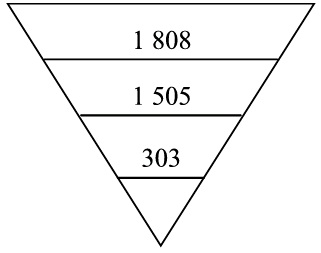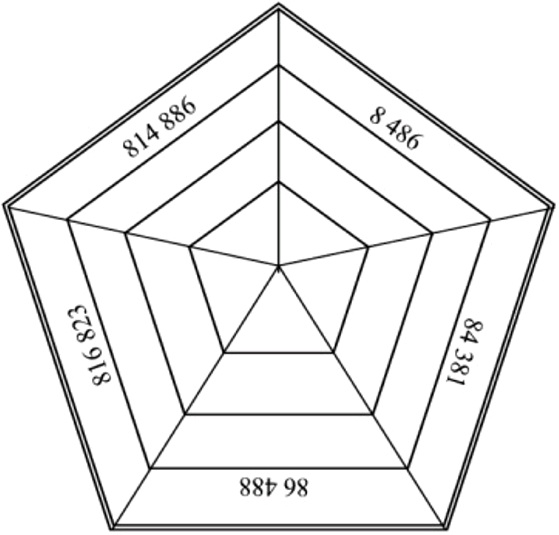e.g.

Figure 1.1.

Figure 1.2.

2.2 What is the difference between the biggest and the smallest answer?

_____________________________________________________________________

2.3 Compare your answers with those of your partner.

2.4 Check your answers with the help of a pocket calculator.

3. What follows is still another technique to calculate the difference between two numbers.

3.1 Work in groups of three again. Read the following problems attentively and explain the

solutions to one another.

a) Kayla's father buys a brand new Renault Scenic for R147 293 and Wayne's father buys a

second-hand car for R69 475. How much more does Kayla’s father pay than Wayne's father?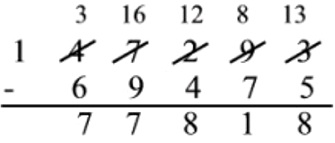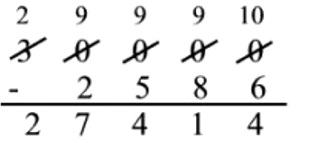Figure 1.3.

Kayla's father pays R77 818 more.

b) Roberto frequently has to make overseas phone calls and his telephone account comes to R30

000. Carli uses her cell phone to make telephone calls and her account comes to R2 586. By what amount is Carli's account less than Roberto's account?

Figure 1.4.

Carli pays R27 414 less.

3.2 Calculate the following by making use of the above method:

a) __________= 42 063 – 19 537

_____________________________________________________________________

_____________________________________________________________________

_____________________________________________________________________

_____________________________________________________________________

b) __________= 264 136 – 198 358

_____________________________________________________________________

_____________________________________________________________________

_____________________________________________________________________

_____________________________________________________________________

c) __________= 900 000 – 231 456

_____________________________________________________________________

_____________________________________________________________________

_____________________________________________________________________

_____________________________________________________________________

TIME FOR SELF-ASSESSMENT

We have now studied different techniques / strategies to do and to check calculations. How do

YOU feel about the work we have just completed?

Assess yourself by circling the figure in the applicable column.

Table 1.2.

COMPLETELY

SLIGHTLY

FAIRLY

VERY

**

UNCERTAIN

CERTAIN

CERTAIN

CERTAIN

I can round off correctly to the

1

2

3

4

nearest 1 000

I can subtract by adding

1

2

3

4

I can estimate the difference by

1

2

3

4

means of rounding off

I can subtract by rearranging

1

2

3

4

numbers in extended notation

I can work with negative numbers

1

2

3

4

I can subtract vertically

1

2

3

4

Puzzle!

You may only use the following keys of your calculator:

1 ; – ; 0 ; =

See if you are able to change the first number into the second

number!

e.g. Change 1 416 to 285

1 416 – 1 110 = 306 – 11 = 295 – 10 = 285

a) Change 378 into 147

_____________________________________________________________________

b) Change 2 598 into 266

_____________________________________________________________________

c) Change 14 210 into 14 000

_____________________________________________________________________

Assessment

Learning Outcome 1: The learner will be able to recognise, describe and represent numbers and their relationships, and to count, estimate, calculate and check with competence and confidence in solving problems.

Assessment Standard 1.10: We know this when the learner uses a range of techniques to perform written and mental calculations with whole numbers;

1.10.1 adding and subtracting in columns.

Assessment Standard 1.11: We know this when the learner uses a range of strategies to check solutions and judge the reasonableness of solutions.

1.5. To solve problems in context*

MATHEMATICS

Number Concept, Addition and Subtraction

Subtraction

EDUCATOR SECTION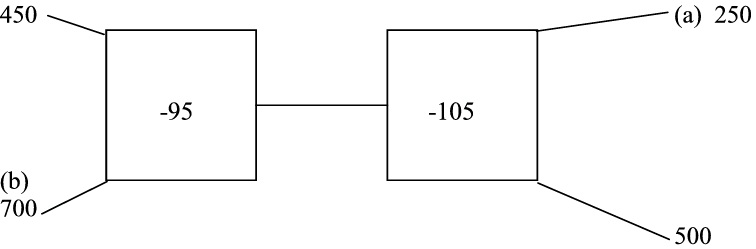Memorandum

Own opinion

TEST 3 TOTAL: 20

1. (a) Difference

1. Addition

2. Minuend

2. (a) 105; 70;

(b) 480; 360;

Figure 1.5.

4. 46 – 000 – 23 000 = 23 000

5. 1 450

6. 23 600 + 400 = 24 000

24 000 + 32 123 = 56 123

32 123 + 400 + 31 = 32 554

7.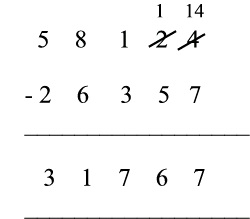Figure 1.6.

8. R500 000 – R13 401

R486 599

Leaner Section

Content

Activity: To solve problems in context [LO 1.6]

CHALLENGE

Here is an assignment for your portfolio. Your educator will go through it with you and explain exactly what is expected of you. Make sure that you understand completely before you begin.

Remember to work neatly!

A challenge!

You have won a competition and are richer by a million rand! But you may only buy articles

advertised in your local newspaper. Cut out the pictures/advertisements of what you would buy

and paste them below, together with the prices. Indicate how much money you will have left after making your purchases. Ask a friend to check your calculations.

ASSESSMENT: ACTIVITY 3.11

Table 1.3.

1

2

3

4

Neatness

Work is untidy Organised,

Neat, well

Neat and organised, easily

and

and un

but hardly

organised and

legible

organisation organised

legible

easily legible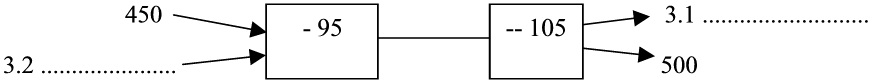Logical

Hardly any

A little logic

Uses effective Mathematical Uses refined logic

reasoning

proof of logic

present

reasoning

and reasoning

Correctness

Answers wrong A few errors

Sufficient understanding, but

of

Answer is correct

(items omitted) committed

subtraction errors committed

calculations

TEST

SEE HOW WELL YOU CAN COPE!

1. Write down the correct answer:

1.1 The answer of a subtraction sum is known as the _______________________